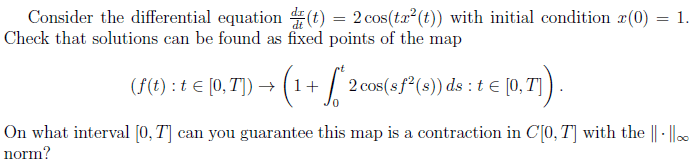# Lipschitz continuousThis question is about lipschitz continuous, i think the way to check if the solutions can be found as fixed points is just differentiating f(t), but i'm not sure about this. Can anyone give me some hints please? I will really appreciate if you can give me some small hints.

## Answers and Replies

You have a map $\Theta:\mathcal{C}([0,T],\mathbb{R})\rightarrow \mathcal{C}([0,T],\mathbb{R})$ such that

$$\Theta(f):[0,T]\rightarrow \mathbb{R}:t\rightarrow 1+\int_0^t 2\cos(sf^2(s))ds$$

Strictly speaking, you first need to check that $\Theta(f)$ is in fact continuous before you can say that the codomain of $\Theta$ is $\mathcal{C}([0,T],\mathbb{R})$.

Now, you need to find out when $\Theta$ is a contraction. Can you tell us what that means??